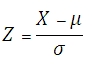# SQL Server STANDARDIZE function

Updated: 9 August 2010

Use STANDARDIZE to calculate a normalized value from a distribution characterized by mean and standard deviation:Syntax
SELECT [wctStatistics].[wct].[STANDARDIZE] (
<@X, float,>
,<@Mean, float,>
,<@Standard_dev, float,>)
Arguments
@X
is the value to be normalized. @X is an expression of type float or of a type that can be implicitly converted to float
@Mean
is the arithmetic mean of the distribution. @Mean is an expression of type float or of a type that can be implicitly converted to float
@Standard_dev
is the standard deviation of the distribution. @Standard_dev is an expression of type float or of a type that can be implicitly converted to float
Return Types
float
Remarks
·         If @Standard_dev ≤ 0, STANDARDIZE returns an error
Examples

Select
wct.STANDARDIZE(-4.6159,10,5)

This produces the following result

----------------------
-2.92318

(1 row(s) affected)Copyright 2008-2023 Westclintech LLC         Privacy Policy        Terms of Service